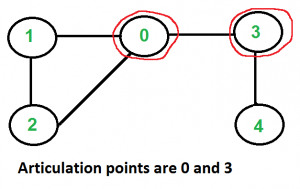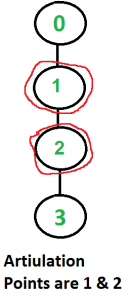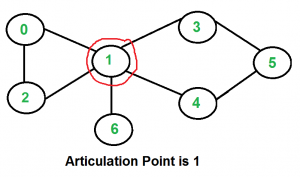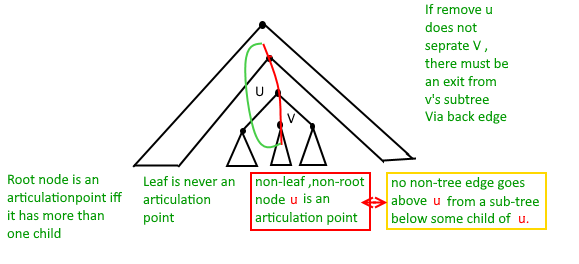Articulation Points (or Cut Vertices) in a Graph

• Difficulty Level : Hard
• Last Updated : 19 Jan, 2022

A vertex in an undirected connected graph is an articulation point (or cut vertex) if removing it (and edges through it) disconnects the graph. Articulation points represent vulnerabilities in a connected network – single points whose failure would split the network into 2 or more components. They are useful for designing reliable networks.

For a disconnected undirected graph, an articulation point is a vertex removing which increases number of connected components.

Following are some example graphs with articulation points encircled with red color.How to find all articulation points in a given graph?
A simple approach is to one by one remove all vertices and see if removal of a vertex causes disconnected graph. Following are steps of simple approach for connected graph.
1) For every vertex v, do following
…..a) Remove v from graph
..…b) See if the graph remains connected (We can either use BFS or DFS)
…..c) Add v back to the graph
Time complexity of above method is O(V*(V+E)) for a graph represented using adjacency list. Can we do better?

A O(V+E) algorithm to find all Articulation Points (APs)
The idea is to use DFS (Depth First Search). In DFS, we follow vertices in tree form called DFS tree. In DFS tree, a vertex u is parent of another vertex v, if v is discovered by u (obviously v is an adjacent of u in graph). In DFS tree, a vertex u is articulation point if one of the following two conditions is true.
1) u is root of DFS tree and it has at least two children.
2) u is not root of DFS tree and it has a child v such that no vertex in subtree rooted with v has a back edge to one of the ancestors (in DFS tree) of u.

Following figure shows same points as above with one additional point that a leaf in DFS Tree can never be an articulation point.We do DFS traversal of given graph with additional code to find out Articulation Points (APs). In DFS traversal, we maintain a parent[] array where parent[u] stores parent of vertex u. Among the above mentioned two cases, the first case is simple to detect. For every vertex, count children. If currently visited vertex u is root (parent[u] is NIL) and has more than two children, print it.

How to handle second case? The second case is trickier. We maintain an array disc[] to store discovery time of vertices. For every node u, we need to find out the earliest visited vertex (the vertex with minimum discovery time) that can be reached from subtree rooted with u. So we maintain an additional array low[] which is defined as follows.

low[u] = min(disc[u], disc[w])
where w is an ancestor of u and there is a back edge from
some descendant of u to w.

Following is the implementation of Tarjan’s algorithm for finding articulation points.

Javascript



Output:

Articulation points in first graph
0 3
Articulation points in second graph
1 2
Articulation points in third graph
1

Time Complexity: The above function is simple DFS with additional arrays. So time complexity is same as DFS which is O(V+E) for adjacency list representation of graph.

References:
https://www.cs.washington.edu/education/courses/421/04su/slides/artic.pdf
http://www.slideshare.net/TraianRebedea/algorithm-design-and-complexity-course-8
http://faculty.simpson.edu/lydia.sinapova/www/cmsc250/LN250_Weiss/L25-Connectivity.htm